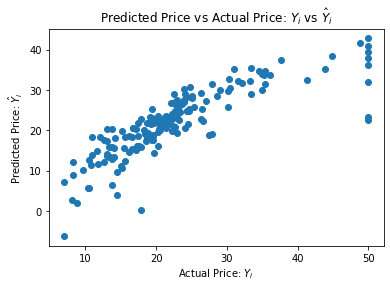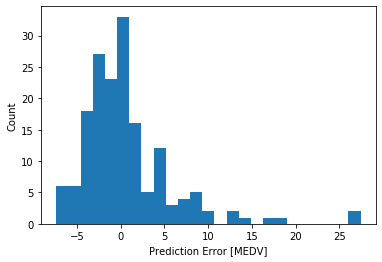# An Example Using Boston House Prices Dataset¶

### Overview¶

Linear regression is one of the most commonly used statistical modeling technique. While not as sexy as machine learning algorithms such as neural networks, it is one of the staple methods used throughout industry. Any card-carrying predictive modeler needs to know this one.

I won't go over the theory of linear regression. Instead, I will reference a few resources that do a good job of describing it.

We will learn a few important things in this simple example, including:

• How to load a standard dataset from sklearn library into a data object (specifically, a data frame)
• How to explore and plot the data we just loaded
• How to fit a linear trend line to the data using OLS

### Data Exploration¶

First we import a the boston house prices dataset, and print a description of it so we can examine what is in the data. Remember in order to execute a 'cell' like the one below, you can 1) click on it and run it using the run button above or 2) click in the cell and hit shift+enter.

In :
import pandas as pd
print(boston.data.shape) #get (numer of rows, number of columns or 'features')
print(boston.DESCR) #get a description of the dataset

(506, 13)
.. _boston_dataset:

Boston house prices dataset
---------------------------

**Data Set Characteristics:**

:Number of Instances: 506

:Number of Attributes: 13 numeric/categorical predictive. Median Value (attribute 14) is usually the target.

:Attribute Information (in order):
- CRIM     per capita crime rate by town
- ZN       proportion of residential land zoned for lots over 25,000 sq.ft.
- INDUS    proportion of non-retail business acres per town
- CHAS     Charles River dummy variable (= 1 if tract bounds river; 0 otherwise)
- NOX      nitric oxides concentration (parts per 10 million)
- RM       average number of rooms per dwelling
- AGE      proportion of owner-occupied units built prior to 1940
- DIS      weighted distances to five Boston employment centres
- TAX      full-value property-tax rate per $10,000 - PTRATIO pupil-teacher ratio by town - B 1000(Bk - 0.63)^2 where Bk is the proportion of blacks by town - LSTAT % lower status of the population - MEDV Median value of owner-occupied homes in$1000's

:Missing Attribute Values: None

:Creator: Harrison, D. and Rubinfeld, D.L.

This is a copy of UCI ML housing dataset.
https://archive.ics.uci.edu/ml/machine-learning-databases/housing/

This dataset was taken from the StatLib library which is maintained at Carnegie Mellon University.

The Boston house-price data of Harrison, D. and Rubinfeld, D.L. 'Hedonic
prices and the demand for clean air', J. Environ. Economics & Management,
vol.5, 81-102, 1978.   Used in Belsley, Kuh & Welsch, 'Regression diagnostics
...', Wiley, 1980.   N.B. Various transformations are used in the table on
pages 244-261 of the latter.

The Boston house-price data has been used in many machine learning papers that address regression
problems.

.. topic:: References

- Belsley, Kuh & Welsch, 'Regression diagnostics: Identifying Influential Data and Sources of Collinearity', Wiley, 1980. 244-261.
- Quinlan,R. (1993). Combining Instance-Based and Model-Based Learning. In Proceedings on the Tenth International Conference of Machine Learning, 236-243, University of Massachusetts, Amherst. Morgan Kaufmann.


In :
# Next, we load the data into a 'dataframe' object for easier manipulation, and also print the first few rows in order to examine it
data = pd.DataFrame(boston.data, columns=boston.feature_names)
data.head() #notice that the target variable (MEDV) is not included

Out:
CRIM ZN INDUS CHAS NOX RM AGE DIS RAD TAX PTRATIO B LSTAT
0 0.00632 18.0 2.31 0.0 0.538 6.575 65.2 4.0900 1.0 296.0 15.3 396.90 4.98
1 0.02731 0.0 7.07 0.0 0.469 6.421 78.9 4.9671 2.0 242.0 17.8 396.90 9.14
2 0.02729 0.0 7.07 0.0 0.469 7.185 61.1 4.9671 2.0 242.0 17.8 392.83 4.03
3 0.03237 0.0 2.18 0.0 0.458 6.998 45.8 6.0622 3.0 222.0 18.7 394.63 2.94
4 0.06905 0.0 2.18 0.0 0.458 7.147 54.2 6.0622 3.0 222.0 18.7 396.90 5.33
In :
#For some reason, the loaded data does not include the target variable (MEDV), we add it here
data['MEDV'] = pd.Series(data=boston.target, index=data.index)
data.describe() #get some basic stats on the dataset

Out:
CRIM ZN INDUS CHAS NOX RM AGE DIS RAD TAX PTRATIO B LSTAT MEDV
count 506.000000 506.000000 506.000000 506.000000 506.000000 506.000000 506.000000 506.000000 506.000000 506.000000 506.000000 506.000000 506.000000 506.000000
mean 3.613524 11.363636 11.136779 0.069170 0.554695 6.284634 68.574901 3.795043 9.549407 408.237154 18.455534 356.674032 12.653063 22.532806
std 8.601545 23.322453 6.860353 0.253994 0.115878 0.702617 28.148861 2.105710 8.707259 168.537116 2.164946 91.294864 7.141062 9.197104
min 0.006320 0.000000 0.460000 0.000000 0.385000 3.561000 2.900000 1.129600 1.000000 187.000000 12.600000 0.320000 1.730000 5.000000
25% 0.082045 0.000000 5.190000 0.000000 0.449000 5.885500 45.025000 2.100175 4.000000 279.000000 17.400000 375.377500 6.950000 17.025000
50% 0.256510 0.000000 9.690000 0.000000 0.538000 6.208500 77.500000 3.207450 5.000000 330.000000 19.050000 391.440000 11.360000 21.200000
75% 3.677083 12.500000 18.100000 0.000000 0.624000 6.623500 94.075000 5.188425 24.000000 666.000000 20.200000 396.225000 16.955000 25.000000
max 88.976200 100.000000 27.740000 1.000000 0.871000 8.780000 100.000000 12.126500 24.000000 711.000000 22.000000 396.900000 37.970000 50.000000
In :
#Load the independent variables (the x1, x2, etc.) into a dataframe object called 'X'. Similarly for the dependent variable 'Y'
X = data.drop('MEDV', axis = 1) #define independent predictor set (excluding the dependent variable)
Y = data['MEDV'] #define the target values (i.e. the dependent variable)


We randomly select a third of our data to be the 'test' dataset. This way we can train our model on 2/3 of the data, and test it on the remainder. Once we are confident that our model is generalizing well (i.e. there is not a HUGE different in the training/testing performance, or in other words, not obviously overfitting), then we can use all of our data to train the model.

In :
from sklearn.model_selection import train_test_split
X_train, X_test, Y_train, Y_test = train_test_split(X, Y, test_size = 0.33, random_state = 5)
print(X_train.shape)
print(X_test.shape)
print(Y_train.shape)
print(Y_test.shape)

(339, 13)
(167, 13)
(339,)
(167,)


### Basic Linear Regression¶

In linear regression we assume that the relationship between the independent variables (X) and the dependent variable (Y) is linear and then go about finding one that minimizes the squared error between the predicted Y and the actual Y.

$${y}_i = \beta_0 + \beta_1 {x}_i + \epsilon_i$$

We now import the LinearRegression method from the sklearn library. Note that the process of creating the model involves the very simple command lm.fit(X,Y). This runs the model and we find the intercept-term, $\beta_0$, and the coefficient $\beta_1$ that minimizes the squared errors.

In :
from sklearn.linear_model import LinearRegression
lm = LinearRegression()
lm.fit(X_train,Y_train)
Y_pred_train = lm.predict(X_train) #predictions on training data
Y_pred = lm.predict(X_test) #predictions on testing data
# We plot predicted Y (y-axis) against actual Y (x-axis). Perfect predictions will lie on the diagonal. We see the diagonal trend, suggesting a 'good' fit
import matplotlib.pyplot as plt
plt.scatter(Y_test,Y_pred)
plt.xlabel("Actual Price: $Y_i$")
plt.ylabel("Predicted Price: $\hat{Y}_i$")
plt.title("Predicted Price vs Actual Price: $Y_i$ vs $\hat{Y}_i$")
plt.show()In :
#Let's get the coefficients
print('Intercept term: ',lm.intercept_) # This gives us the intercept term
print('Coefficients: \n',lm.coef_) # This gives us the coefficients (in the case of this model, just one coefficient)

Intercept term:  32.8589326340861
Coefficients:
[-1.56381297e-01  3.85490972e-02 -2.50629921e-02  7.86439684e-01
-1.29469121e+01  4.00268857e+00 -1.16023395e-02 -1.36828811e+00
3.41756915e-01 -1.35148823e-02 -9.88866034e-01  1.20588215e-02
-4.72644280e-01]


We can inspect a few key values such as the coefficient and the mean-squared-error by executing the cell below.

In :
from sklearn.metrics import mean_squared_error, mean_absolute_error, r2_score
mse = mean_squared_error(Y_test,Y_pred)
print('Mean Squared Error: ',mse)
mae = mean_absolute_error(Y_test,Y_pred)
print('Mean Absolute Error: ',mae)
rsq = r2_score(Y_train,Y_pred_train) #R-Squared on the training data
print('R-square, Training: ',rsq)
rsq = r2_score(Y_test,Y_pred) #R-Squared on the testing data
print('R-square, Testing: ',rsq)

Mean Squared Error:  28.530458765974583
Mean Absolute Error:  3.4550349322483482
R-square, Training:  0.7551332741779997
R-square, Testing:  0.6956551656111607


We can get a distribution of the error, below.

In :
error = Y_test - Y_pred
plt.hist(error, bins = 25)
plt.xlabel("Prediction Error [MEDV]")
_ = plt.ylabel("Count")### Feedback¶

If you have ideas on how to improve this post, please let me know: https://predictivemodeler.com/feedback/